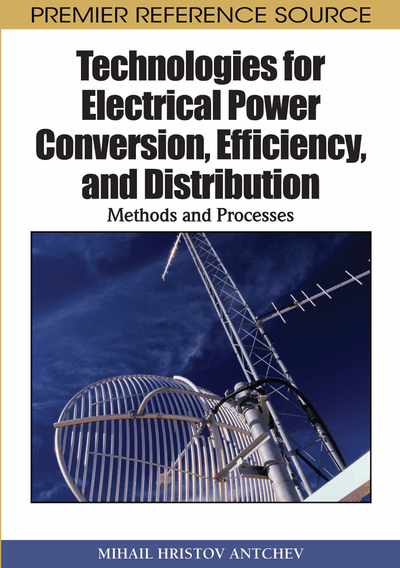# DC/DC Conversion

DOI: 10.4018/978-1-61520-647-6.ch006
Available
\$37.50
No Current Special Offers

## Abstract

The basic idea of converting DC into DC voltages has been studied in Section 1 Chapter 3. There also has been mentioned that to separate DC component a smoothing filter is connected between the output of the converter and the load. The filter consists of passive elements – inductances and capacitors, and in several cases it is included in the converters circuit (Williams, 2008). Figure 1 displays the waveform of the output voltage as the block of the converter contains and the smoothing filter. Using the figure the basic indicators of the converter will be defined.
Chapter Preview
Top

## Basic Indicators

The basic idea of converting DC into DC voltages has been studied in Section 1 Chapter 3. There also has been mentioned that to separate DC component a smoothing filter is connected between the output of the converter and the load. The filter consists of passive elements – inductances and capacitors, and in several cases it is included in the converters circuit (Williams, 2008). Figure 1 displays the waveform of the output voltage as the block of the converter contains and the smoothing filter. Using the figure the basic indicators of the converter will be defined.

Efficiency coefficient of the converter is the ratio of the output to the input powers:

(6.1)

This coefficient should be as high as possible.

Coefficient of the ripples of the output voltage is defined analogue to those in the rectifiers (see Chapter 4):, (6.2) where inis the maximum value of the first harmonic of the output voltage.

The right part of the equation is obtained on the assumption that the first harmonic is a sinusoidal function which positive amplitude coincides with the maximum value of the ripples shown in Figure 1, and which negative amplitude coincides with the minimum value of the ripples also shown in Figure 1. This coefficient should be as low as possible.

Output characteristics also exist in DC/DC converters like those in the rectifiers (see Chapter 4). These characteristics give the change of the output voltage UOUT at the change of the output current IOUT.

Top

## Conversion Without Galvanic Isolation

The following converters without galvanic isolation are known – buck, boost, buck/boost, Cuk converter, Single-Ended Primary Inductance Converter (SEPIC) (Billings, 1999), (Kazimierczuk, 2008).

Figure 2 shows the electrical schematic of and the waveforms explaining the operation of a buck converter. It may operate in two modes – discontinuous-conduction and continuous-conduction current modes through the inductance. A fully-controlled power device able to turn on and off by a control electrode is shown using the circuit symbol for a switch in the figure. The switch can be a power BJT, power MOSFET or IGBT. Ratio of the pulse time (time during which the switch conducts) to the operation period defines duty ratio. It is important for the converter operation and it is always less than 1. When the transistor is on, the current through the inductance increases and recharges the capacitor. This is so-called forward motion; since during it energy is passed to the output capacitor, the converter is frequently called forward converter.

When the transistor is off, the current through the inductance decreases flowing through the diode VD. This is so-called reverse motion, the diode is called a commutation diode. In the case of continuous-conduction mode, the relationship between the output and input voltages is:and(6.3)

In the case of discontinuous-conduction current mode through the inductance, the relationship is:

(6.4)

## Complete Chapter List

Search this Book:
Reset